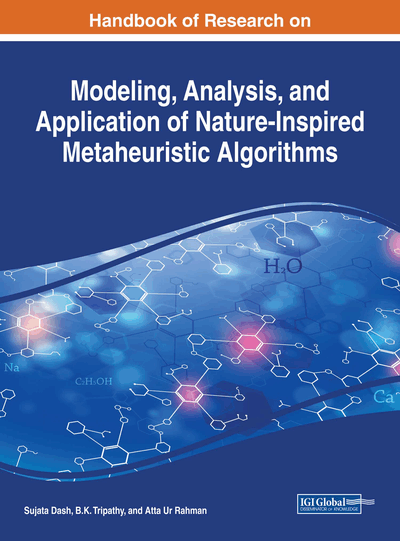# Memetic Algorithms and Their Applications in Computer Science

B. K. Tripathy (VIT University, India), Sooraj T. R. (VIT University, India) and R. K. Mohanty (VIT University, India)
DOI: 10.4018/978-1-5225-2857-9.ch004
Available
\$37.50
No Current Special Offers

## Abstract

The term “memetic algorithm” was introduced by Moscato is an extension of the traditional genetic algorithm. It uses a local search technique to reduce the likelihood of the premature convergence. Memetic algorithms are intrinsically concerned with exploiting all available knowledge about the problem under study. MAs are population-based metaheuristics. In this chapter we explore the applications of memetic algorithms to problems within the domains of image processing, data clustering and Graph coloring, i.e., how we can use the memetic algorithms in graph coloring problems, how it can be used in clustering based problems and how it is useful in image processing. Here, we discuss how these algorithms can be used for optimization problems. We conclude by reinforcing the importance of research on the areas of metaheuristics for optimization.
Chapter Preview
Top

## Introduction

Optimization algorithms can be roughly divided into two categories: Exact algorithms and heuristics. Exact algorithms are designed in such a way that they will find optimum solution in a finite amount of time. However, for very difficult optimization problems (e.g. NP-hard) the finite amount of time may increase exponentially with respect to the dimensions of the problem. Heuristics do not have this guarantee, and therefore generally return solutions that are worse than optimal. However, heuristic algorithms usually find “good” solutions in a “reasonable” amount of time. The best solution cannot be found through heuristics, but heuristics can find a realizable solution with limited time and information. For design optimization using heuristics, however, an acceptable solution can be found, but there is no practical way of determining how close to a global optimum the final design is (Coley and Schukat, 2002).

Many heuristic algorithms are very specific and problem-dependent. On the other hand, a metaheuristic is a high-level problem-independent algorithmic frame-work that provides a set of guidelines or strategies to develop heuristic optimization algorithms. But a concrete definition has been elusive and in practice many researchers and practitioners interchange these terms. Thus, the term metaheuristic is also used to refer to a problem specific implementation of a heuristic optimization algorithm according to the guidelines expressed in such a framework. In contrast to heuristics, meta-heuristics designate a computational method that optimizes a problem by trying iteratively to improve a candidate solution with regard to a given measure of quality. It can search very large option spaces of candidate solutions.

A metaheuristics is an algorithm designed to solve approximately a wide range of hard optimization problems without having to deeply adapt to each problem. Indeed, the Greek prefix ‘‘Meta’’, present in the name, is used to indicate that these algorithms are ‘‘higher level’’ heuristics, in contrast with problem-specific heuristics. Metaheuristics are generally applied to problems for which there is no satisfactory problem-specific algorithm to solve those (Boussaïd et al., 2013).

### Memetic Algorithms

A memetic algorithm is an extension of the traditional genetic algorithm. It uses a local search technique to reduce the likelihood of the premature convergence. The term “memetic algorithm” was introduced by Moscato in his technical report in 1989. Memetic algorithms are intrinsically concerned with exploiting all available knowledge about the problem under study. MAs are population-based metaheuristics. This means that the algorithm maintain a population of solutions for the problem at hand, i.e., a pool comprising several solutions simultaneously. Each of these solutions is termed “individual” in the EA jargon, following the nature-inspired metaphor upon which these techniques are based

Top

## Applications Of Memetic Algorithms

In this section we discuss some of the applications of memetic algorithms.

## Key Terms in this Chapter

GCP: Graph coloring problem is to assign colors to certain elements of a graph subject to certain constraints.

Memetic Algorithm: A memetic algorithm is an extension of the traditional genetic algorithm. It uses a local search technique to reduce the likelihood of the premature convergence.

Meta Heuristic Algorithm: In computer science and mathematical optimization, a metaheuristic is a higher-level procedure or heuristic designed to find, generate, or select a heuristic (partial search algorithm) that may provide a sufficiently good solution to an optimization problem, especially with incomplete or imperfect information or limited computation capacity.

SAR Image: Synthetic aperture radar (SAR) is a form of radar that is used to create images of objects, such as landscapes – these images can be either two or three dimensional representations of the object.

## Complete Chapter List

Search this Book:
Reset